Select Page

Common Core Standard: 7.G.B.4

If area is defined as the number of unit squares that cover the surface of a closed figure, then how does this apply to a circle? Can we remember the abstract formula for area of a circle better by understanding where it comes from visually? Can we understand each permutation of an equation? While working with circles, what can we learn about the meanings of coefficients, linear terms and quadratic terms?  These are the questions this Sticky Math Lesson sets out to answer.

This Sticky Math Lesson examines the area of a circle conceptually by connecting to the area of rectangle / parallelogram. It is helpful to examine the relationship between the area of a rectangle and any parallelogram before this lesson. Incidentally, this lesson is a good example of stopping to contextualize (MP2) in each of the permutations of the area equation. The lesson can introduce vocabulary through the drawings, offers an opportunity for students to use precise vocabulary (MP6), and acts as a formative assessment of academic vocabulary.

While I have used these visuals (in gray) with students to help them understand how the area of a circle relates to the area of a rectangle in the past, this time I challenged myself to represent visually each permutation of the area formula (in black).  Along the way, I deepened my understanding of the meaning of coefficients, linear terms and quadratic terms.  I hope you find it illuminating.

BACKGROUND KNOWLEDGE (WARMUP)

While not necessary, it is nice for students to know the relationship between the area of a parallelogram and the area of a rectangle before exploring the relationship between the area of circle and a rectangle as it is an intermediate step visually.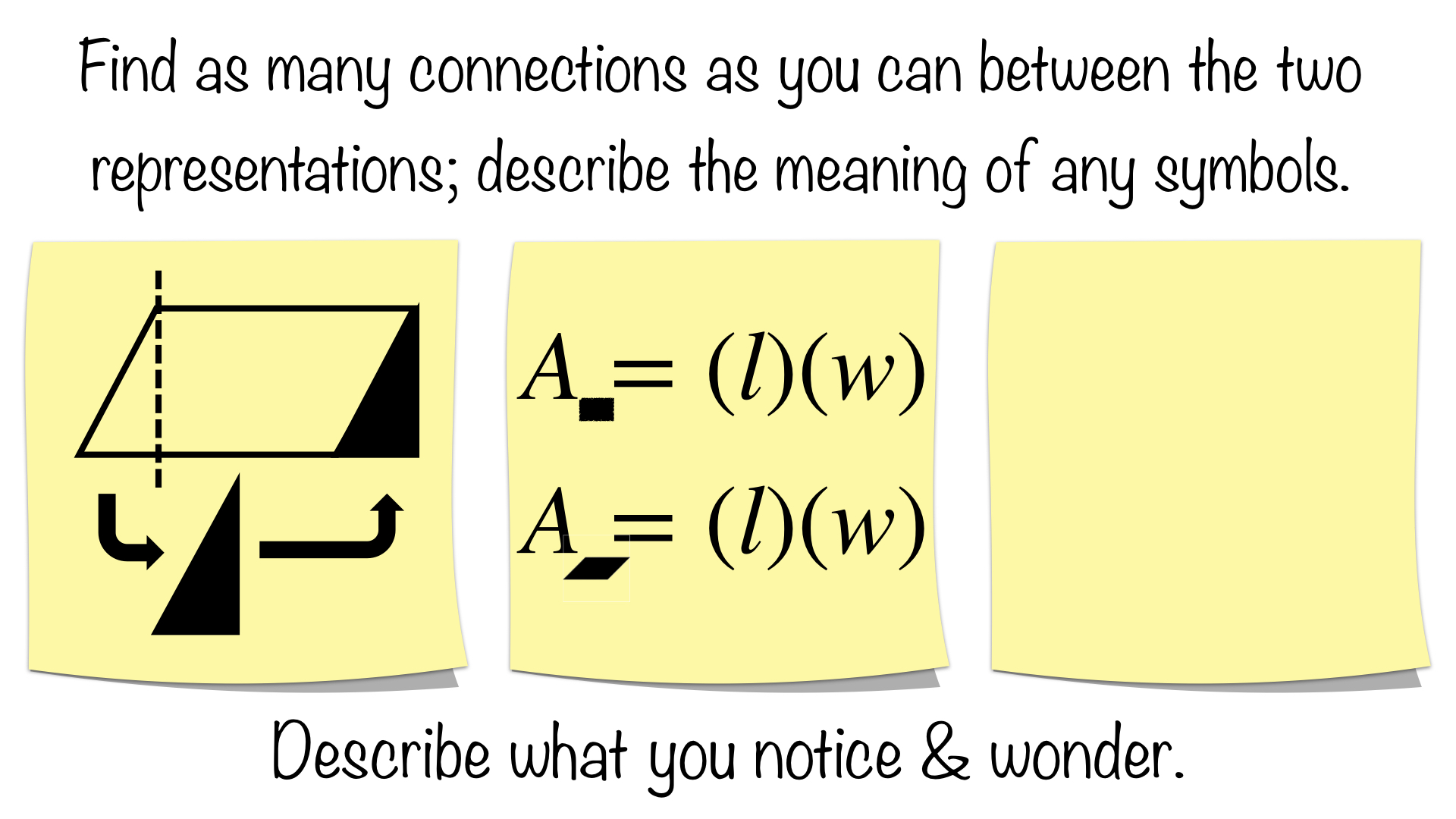Connections could include:

• On the left sticky, if the triangle is “cut” and placed on the opposite side, then the result is a rectangle
• On the right sticky, the formula for the area of each is the same
• The parallelogram and the rectangle have the same value for their widths (or heights)
• The parallelogram and the rectangle have the same value for their lengths (or bases)

Students could create their own representations; these might include:

• Describing the relationship in words
• Drawing the new rectangle
• Rewriting the equations with base and height

The big takeaway here is that the area of a parallelogram can be shown to be equivalent.

STEP #1: AN ALTERNATE VIEW OF THE AREA OF A CIRCLE

Before introducing the abstract, symbolic representation of the area of a circle, we want to be sure students understand what has taken place visually.  One way is to use the Sticky Math Pair below; another is to have students cut out the circle, cut the circle, and then paste it onto their Sticky Math Recording Sheet.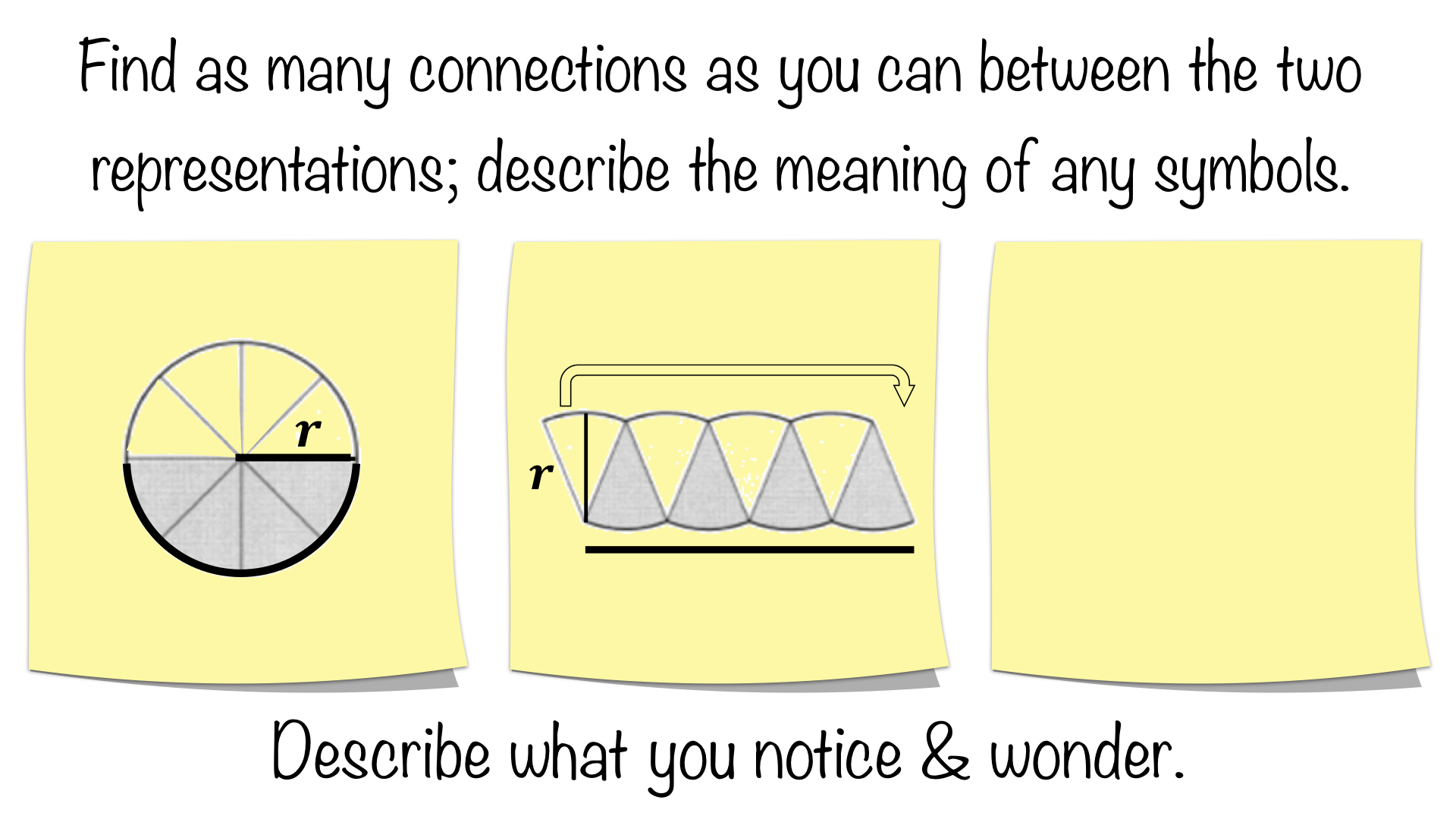Connections could include:

• both stickies represent the the area of a circle

• shading is not representative of the entire area of the circle; it represents half the area of the circle.

• the middle sticky has the area of circle organized so it is similar to a parallelogram, or a rectangle if you think of moving half of an eighth (1 sixteenth).

• the curved line in the left sticky is equivalent to the longer straight line in the middle sticky

• r, the radius of the circle, in the left sticky is the height of the new figure in the middle sticky
• the four gray “slices” in the left sticky make up half of the new figure on the middle sticky
• the four open “slices” in the right sticky make up half of the new figure on the middle sticky
• the longer line is one half of the circumference
• the longer line is the “base” of the new figure
• both figures have the same area
• the arrow on the middle sticky seems to indicate moving part of the left side to the right side

Students could create their own representations; these might include:

• organizing the “slices” differently
• drawing the new figure more like a “rectangle” by cutting an end piece in half (bisecting the angle) and moving it
• writing the formula for area of the circle or even one its halves

Students may not come up with the last two bullets; however, you can introduce them one at a time if you like on individual stickies.

STEP #2: FOCUSING ON AREA BY THE INTRODUCTION OF A FORMULA

You could skip to this Sticky Math Triad to begin, but it is a lot to take in at once without the previous Sticky Math Pair (and you do not want to overwhelm the students’ working memories).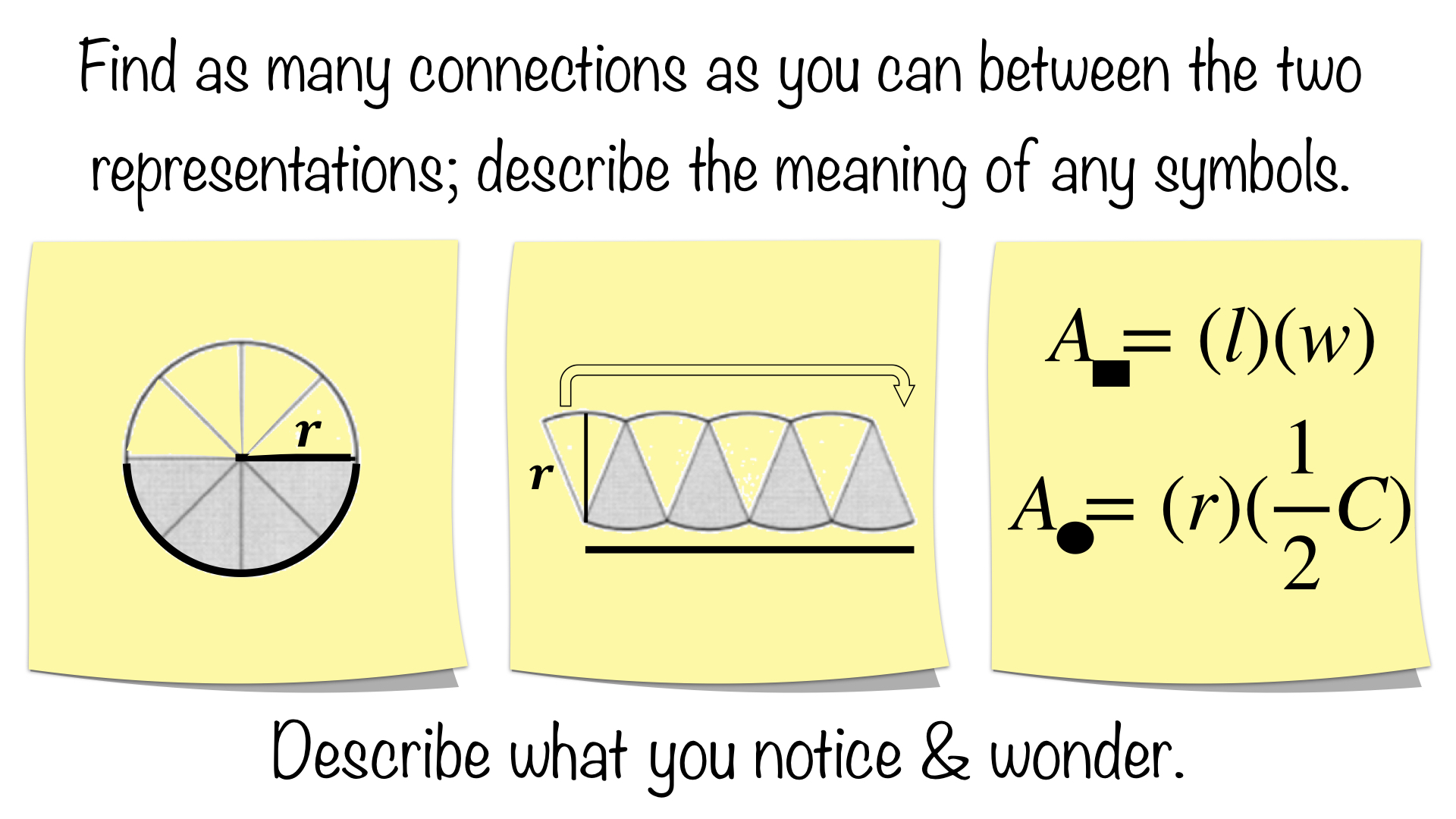Connections between the left and middle stickies were discussed above; additional connections could include:

• all three stickies have representations of the the area of a circle
• half the circumference is found on the bottom portion of the circle on the left sticky, on the “base” of the middle sticky, and in the formula for the area of a circle on the right sticky
• the radius is the r on the left, middle, and right stickies
• the radius is one dimension of the area formula of a rectangle (students may say length or width, base or height)
• half the circumference is another dimension of the area formula of a rectangle (students may say length or width, base or height)
• On the right sticky, the A in the lower formula has a circle by it, it contains radius, and it contains half of a circumference; it refers to the area of a circle
• On the right sticky, the A in the upper formula has a rectangle by it, it contains length, and it contains height; it refers to the area of a rectangle

The key here is to notice that the area of a circle is being defined as its height, the radius, times its “width”, half of a circumference.

At this point, you may have noticed that I have been putting some terms in quotes, mainly the references to the “base”; the reason for which is that it is made up of a group of arcs rather than a straight line.  The is only an informal proof here, but it is worth noting that if you made slices progressively smaller the resulting “rectangular” figure becomes more and more like an actual rectangle.  This could lead to providing some background knowledge in preparation for calculus by exploring some of the ideas behind limits and derivatives.  As the arc lengths become infinitely small, the slope of the tangent line approaches zero or a straight (horizontal) line: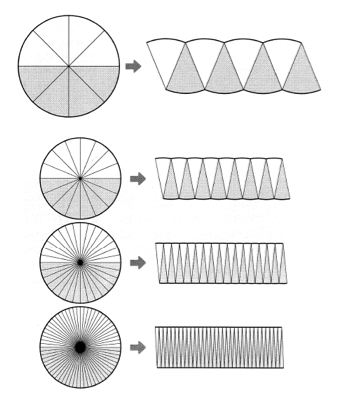STEP #3: FORMULA IN TERMS OF RADIUS

We begin our move toward the commonly used πr^2 by putting our formula in terms of radius.  For more on the visuals that accompany circumference, see my blogpost on Circumference of a Circle and the Meaning of Pi.  Note that added side length to our parallelogram, which allows for the replacement of the full circumference by the equivalent term of 2πr: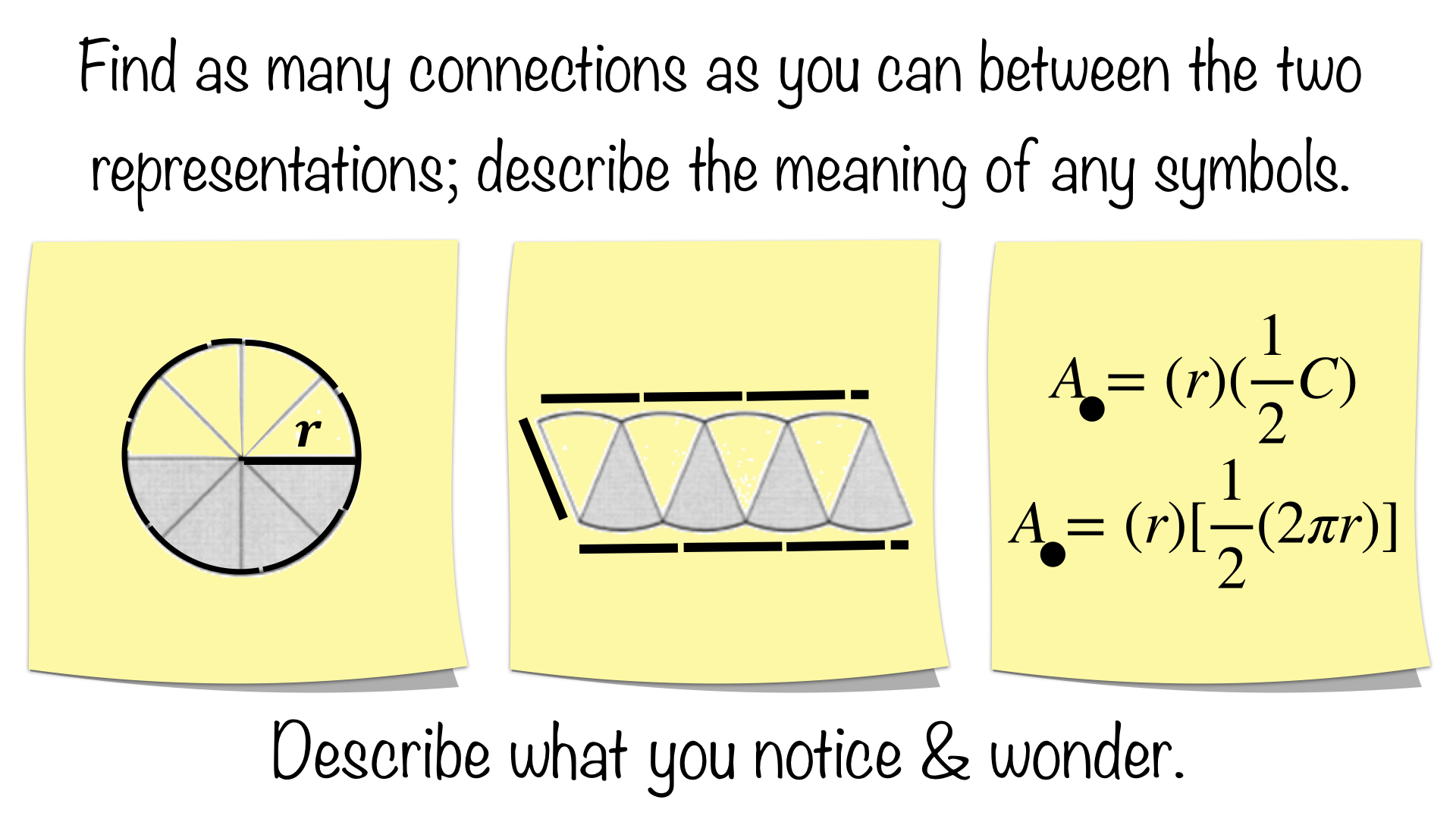Connections could include:

• the arcs on the circumference appear to be equivalent in “length” to the radius
• on the left sticky, the circumference has been replaced by 2π radii or the radius pi times, twice or 2r, pi times
• the circumference of circle on the left sticky is the same as the two “lengths” in the middle sticky
• on the middle sticky, the lines making up the “length” appear to be the same length as the radius
• on the middle sticky, the “length” appears to be three radii and a little bit more…
• on the middle sticky, the “length” is now the radius pi times, which is half the circumference still
• on the right sticky the lower formula is the same as the formula above except the C has been replaced by 2πr

I have said this in other blogposts, but it bears repeating.  It is fine to allow students to restate a connection made previously as this helps the student appropriate someone else’s connection and make it their own.  It also provides a chance to use vocabulary.

STEP #4: SIMPLIFYING THE FORMULA

At this point, we simplifying the formula towards our goal of πr^2; however, the challenge is to do so visually:Connections could include:

• on the left sticky, the formula on top is represented by the middle sticky
• on the left sticky, the formula on the bottom is represented by the right sticky
• the middle sticky looks more like a parallelogram
• the right sticky looks more like a rectangle after you move the half of the eighth over or a sixteenth.
• comparing the middle sticky to the right sticky, the black lines have gone from showing a full circumference (top & bottom) to showing half a circumference only (bottom)
• the r on the left sticky is equivalent to the height on the right sticky
• the πr on the left sticky is equivalent to the “length” on the other stickies
• the “length” on all three is pi times r or stated more conceptually r pi times

We can clearly see we are a single step from our goal of πr^2; however, if we stopped here, then we might not build any understanding about the meaning of a quadratic term.  Before we do so, let’s stop and note the meaning of a linear term.

BONUS EXTENSION: MEANING OF A LINEAR TERM & ITS COEFFICIENT

In order to fully understand the meaning of πr, lets move through levels of abstraction to get there rather than jumping to its full abstraction.  3r is r three times:In this case, we think of the coefficient of 3 as the number of lines and r as the length of the line.

Replacing 3 with a variable of p: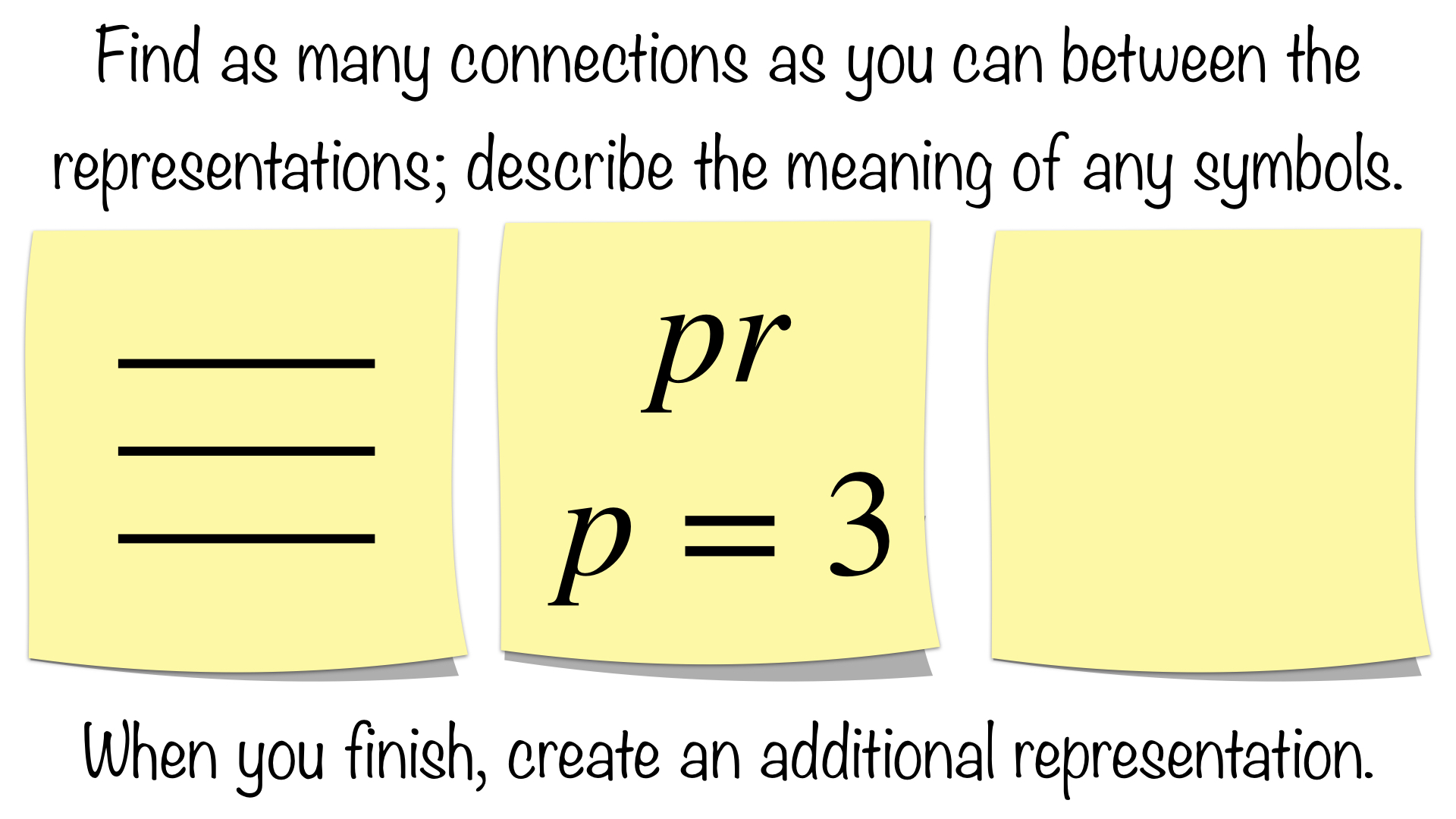In this case, we think of p as the number of lines and r as the length of the line.

Abstracting further, we replace the rational number three with the irrational pi:In this case, we think of pi as the number of lines and r as the length of the line.  Of course, we have taken some liberty of drawing an irrational side length here by approximating it as about 3.14 (yes, the drawing is roughly to scale).

Now back to our final step!

STEP #5 SIMPLIFYING TO PI TIMES R SQUARED

We could just utilize an exponent and be finished, arriving at πr^2, but then we would miss out on deepening understanding of a quadratic term.  Some might argue this is unnecessary in lower grades; however, I might remind them that students are exploring area and substituting into and evaluating quadratic terms in sixth grade.  A quadratic term is two dimensional and basically can be thought of a symbolic notation of area.  Let’s use our current topic as an example as we finally arrive at πr^2.  In other words, we can write πr^2, but what does it look like?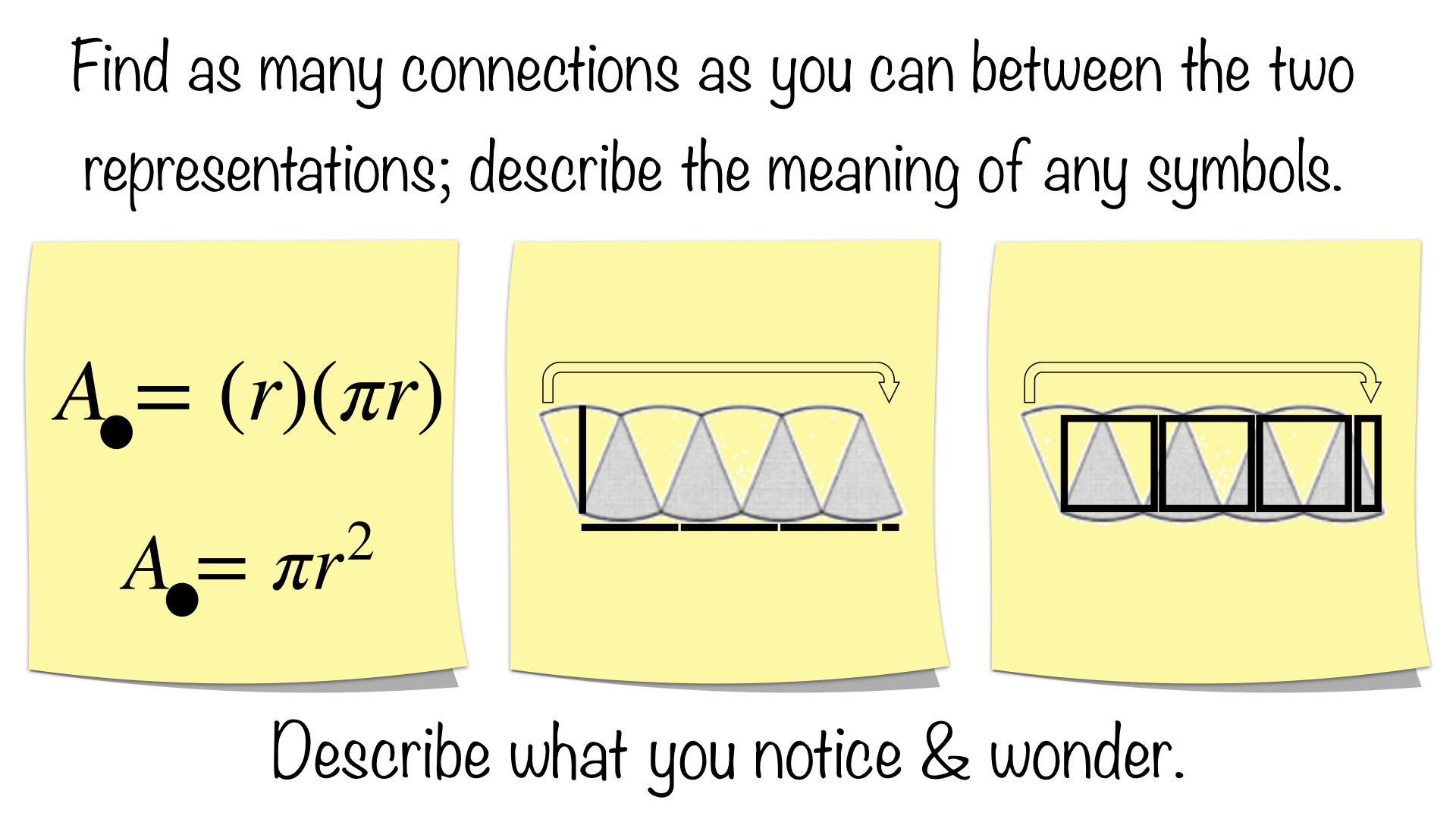Connections could include:

• any previous connection
• on the left sticky, the top formula is represented by the middle sticky
• on the left sticky, the bottom formula is represented by the right sticky
• the top formula and the middle sticky show the factors or dimensions of the figure
• the bottom formula and the right sticky show the product or area of the figure
• the πr in the top formula on the left sticky is the radius pi times along the length of the figure in both of the middle and right stickies
• the length of the sides of the squares is r, the radius
• each square is r^2
• there are 3 radius squares and part of a radius square
• there are pi radius squares or a radius squared, pi times

So we have arrived, finally!  The area of a circle can be reorganized into a figure similar to a parallelogram.  Using length times width or base times height we can see that the area of a circle can be thought of as the radius times one half of its circumference, which can be rewritten as the radius times pi radii or pi times the radius squared.  Notice we also can “see” the area of a circle in terms of square units now.

Why take the time do this though?  While I do not expect students to remember everything found here, I do want to help them remember the formula of πr^2 either remembering the activity or understanding just the final Sticky Math Triad.  After the day long cutting, pasting, labeling, and working through the informal proof; you could revisit this later as a warmup/interleaved practice.  By just using the final Sticky Math Triad or this Pizza Pi example, where you can use the edge crust to talk about circumference and the cheesy-pepperoni goodness to discuss the area:I conclude with the final extension to the meaning of a quadratic term and its coefficient, since it is right in front of us anyway.

BONUS EXTENSION: MEANING OF A QUADRATIC TERM & ITS COEFFICIENT

In order to fully understand the meaning of πr^2, lets move through levels of abstraction to get there rather than jumping to its full abstraction.  3r^2 is r^2 three times: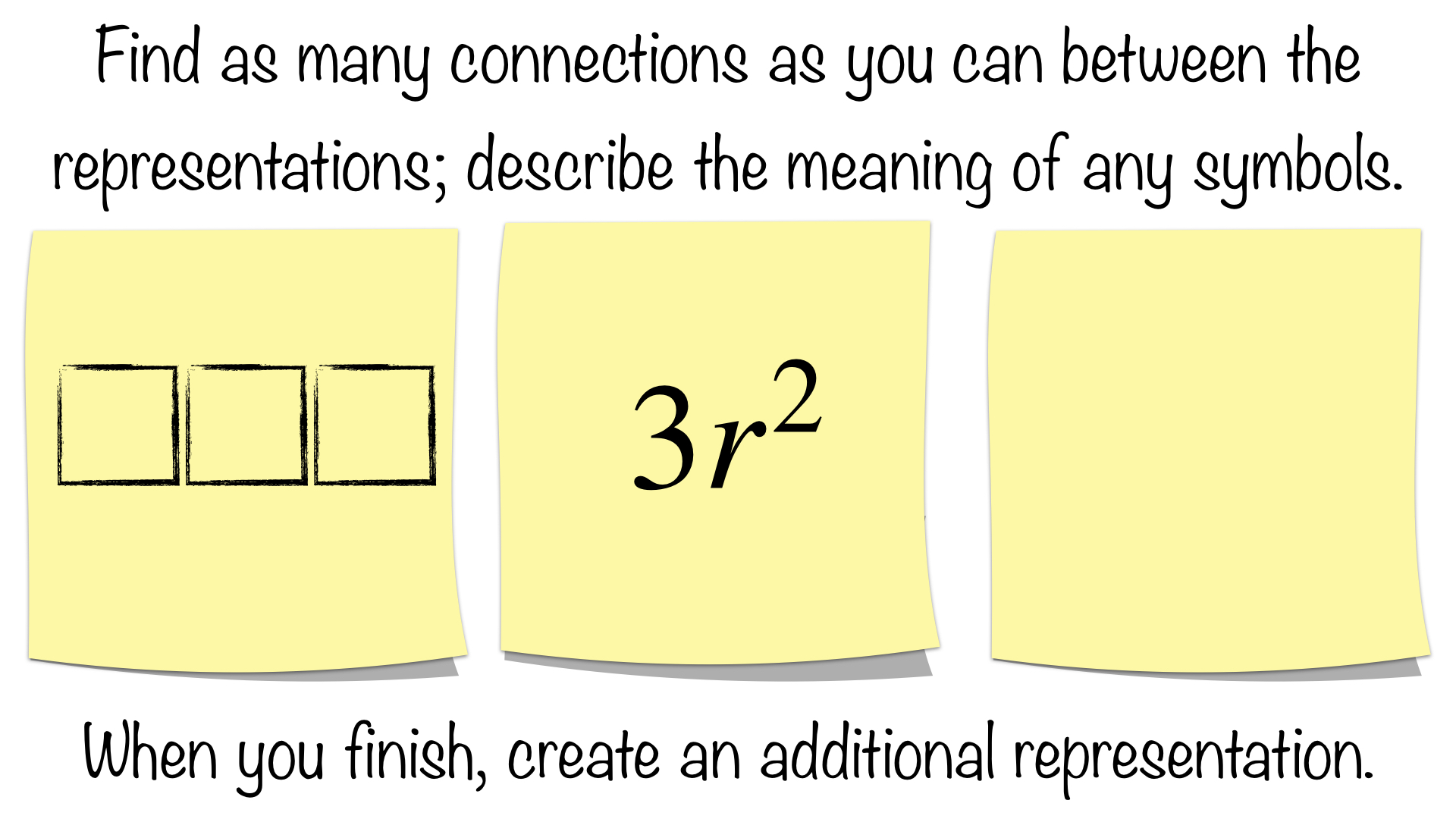In this case, we think of the coefficient of 3 as the number of squares and r^2 as the area of the square.

Replacing 3 with a variable of p: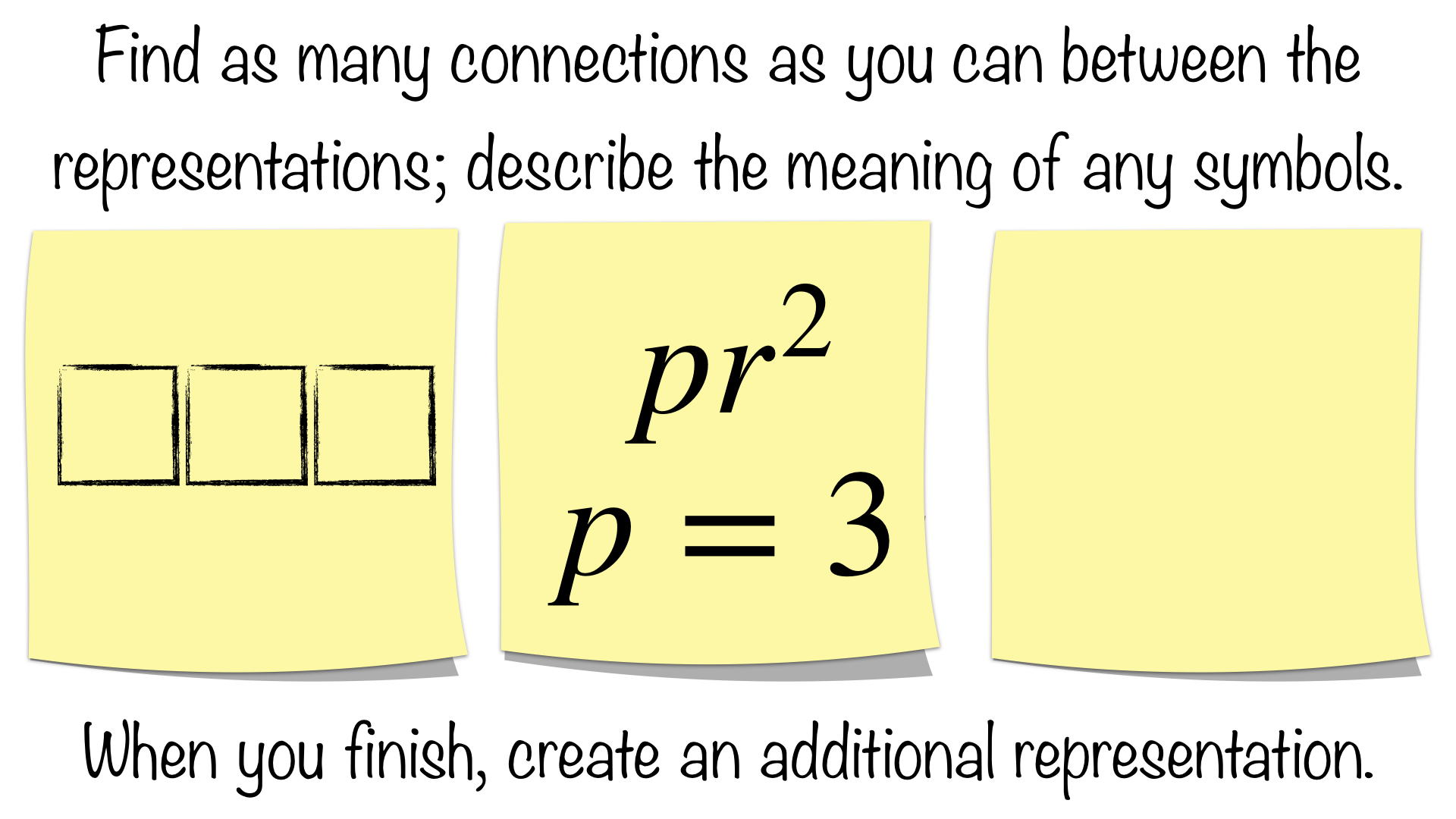In this case, we think of p as the number of squares and r^2 as the area of the square.

Abstracting further, we replace the rational number three with the irrational pi:In this case, we think of pi as the number of squares (even though it is irrational) and r^2 as the area of the square.  Of course, we have taken some liberty of drawing an irrational side length here by approximating it as about 3.14 (yes, the drawing is roughly to scale).

Well, that is about all I can pull out of that!  If you made it this far, then you are to be commended.  Feel free to submit for time spent in professional development.  Just not to me… maybe that will come much later down the road!

Common Core Standards: 7.G.B.4

What connections are your students making?  What modifications are you making to use this with students?  We would love to hear your feedback; please submit a comment below or consider submitting your own Sticky Math activity here.